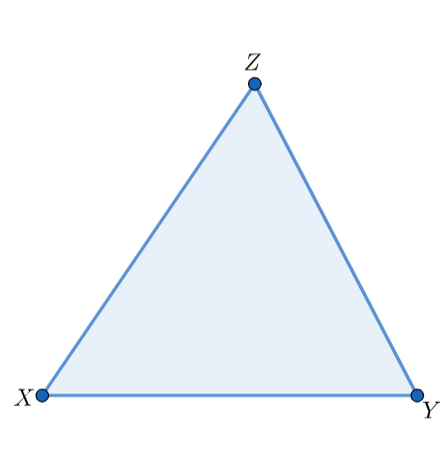Courses
Courses for Kids
Free study material
Free LIVE classes
More# In$\Delta ABC$ , $\measuredangle A={{75}^{o}},\measuredangle B={{65}^{o}}$. Find $\measuredangle C$ .Verified
324.6k+ views
Hint: The given problem is related to the angle sum property of a triangle. The sum of the three interior angles of a triangle is equal to ${{180}^{o}}$ .

We will consider a triangle $\Delta XYZ$. The angles formed by any two sides of the triangle and lying on the inside of the triangle are called internal angles of a triangle. In $\Delta XYZ$, the angles $\measuredangle XYZ,\measuredangle YXZ$ and $\measuredangle XZY$ are the internal angles.The angle sum property of a triangle states that the sum of internal angles of a triangle will always be equal to ${{180}^{o}}$. This property is valid for all the triangles. It is a necessary (but not sufficient) condition for a polygon to be a triangle. If the sum of internal angles of a polygon is not equal to ${{180}^{o}}$, then that polygon can never be a triangle. But the inverse is not necessarily true, i.e. if the sum of internal angles of a polygon is equal to ${{180}^{o}}$ , then it is not necessary that the polygon is a triangle. According to the angle sum property of a triangle, $\measuredangle XYZ+\measuredangle YXZ+\measuredangle XZY={{180}^{o}}$ .
Now, the triangle given in the question is $\Delta ABC$ . It is also given that the measures of angles $\measuredangle A$ and $\measuredangle B$ are ${{75}^{o}}$and ${{65}^{o}}$ respectively. According to the angle sum property of a triangle, the sum of internal angles of $\Delta ABC$ is equal to ${{180}^{o}}$, i.e. $\measuredangle A+\measuredangle B+\measuredangle C={{180}^{o}}$ . But we are given that $\measuredangle A={{75}^{o}}$ and $\measuredangle B={{65}^{o}}$. So, ${{75}^{o}}+{{65}^{o}}+\measuredangle C={{180}^{o}}$ .
$\Rightarrow {{140}^{o}}+\measuredangle C={{180}^{o}}$
$\Rightarrow \measuredangle C={{180}^{o}}-{{140}^{o}}={{40}^{o}}$
So, the measure of $\measuredangle C$ is equal to ${{40}^{o}}$.

Note: Always make sure that calculation mistakes are not present while solving the questions. Generally, students make calculation mistakes while solving a problem in a hurry. So, try to avoid calculation mistakes.

Last updated date: 30th May 2023
Total views: 324.6k
Views today: 8.83k